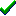# fg_texmapp()Win32DirectXFastgraph/Light

Prototype

void fg_texmapp (int *vertex_array, void *uv_array, double *xyz_array, int n);

Sub fg_texmapp (vertex_array() As Long, uv_array() As Any, xyz_array() As Double, ByVal n As Long)

procedure fg_texmapp (var vertex_array : integer; var uv_array; var xyz_array : double; n : integer);

Description

The fg_texmapp() function draws a projected perspective corrected texture-mapped convex polygon in screen space, with 2D clipping and automatic backface removal. This function is called internally by Fastgraph's 3D functions and is not usually called directly by applications.

Parameters

vertex_array is the name of the array containing the (x,y) coordinate pairs of each polygon vertex. The first array element is the x component of the first vertex, the second element is the y component of the first vertex, the third element is the x component of the second vertex, and so forth. The vertices must be stored in clockwise order, meaning you would travel clockwise along the polygon edge to go from one vertex to the next.

uv_array is the name of the array containing the (u,v) texture map coordinates for each (x,y) coordinate pair in vertex_array. The first two uv_array elements represent the (x,y) values at the first vertex in vertex_array, the next two uv_array elements are for the second vertex, and so forth. The fg_tmunits() function defines if uv_array contains integer or 32-bit floating point values.

xyz_array is the name of the array containing the 3D (x,y,z) coordinates for each (x,y) coordinate pair in vertex_array. The first three xyz_array elements represent the (x,y,z) values at the first vertex in vertex_array, the next three xyz_array elements are for the second vertex, and so forth. Only the z coordinates are meaningful in this function.

n is the number of vertices in each of the above arrays.

Return value

none

Restrictions

If you attempt to fill a non-convex polygon with fg_texmapp(), or if the vertices are not stored in clockwise order, only a portion of the polygon will be filled.

See also

Contents
Fastgraph Home Page

copyright 2001 Ted Gruber Software, Inc.